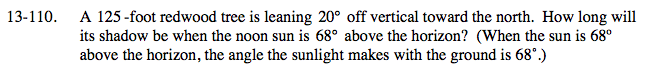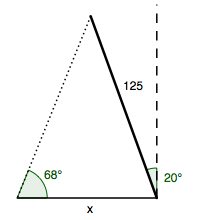### Home > A2C > Chapter Ch13 > Lesson 13.2.2 > Problem13-110

13-110.

A 125 -foot redwood tree is leaning 20° off vertical toward the north. How long will its shadow be when the noon sun is 68° above the horizon? (When the sun is 68° above the horizon, the angle the sunlight makes with the ground is 68°.) Homework Help ✎Draw a diagram representing the situation.Use angle relationships and the Law of Sines to solve for x.

90° − 20° = 70°
180° − 70° − 68° = 42°

$\frac{\text{sin}168\degree}{125}=\frac{\text{sin}42\degree}{x}$

x ≈ 90.21 feet